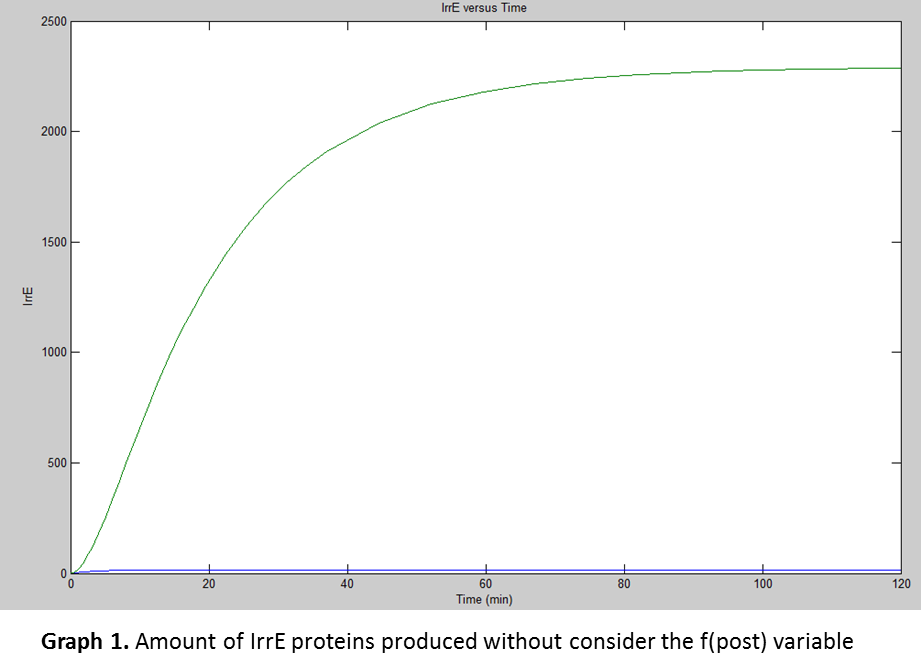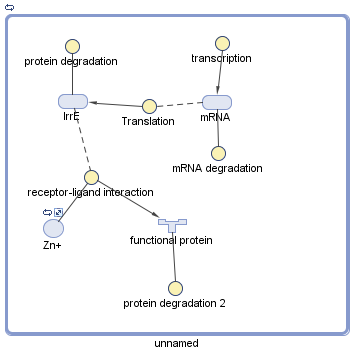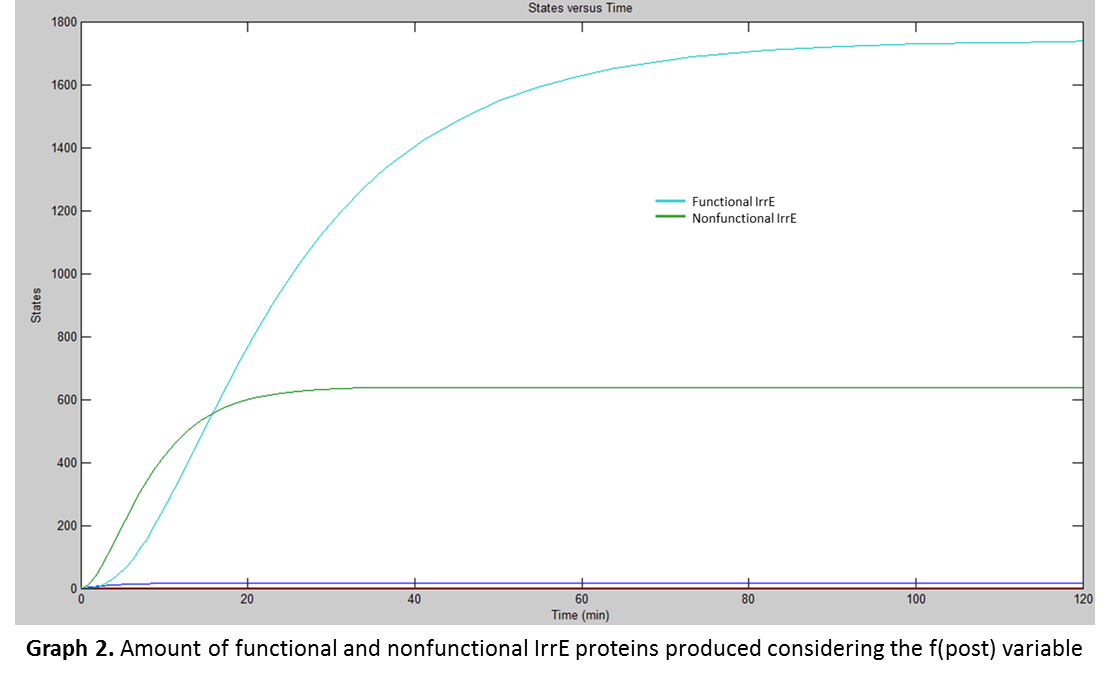# Team:CIDEB-UANL Mexico/math resistance

iGEM CIDEB 2014 - Project

## Resistance Module

The resistance module is based in the use of IrrE. IrrE is a protein which is not affected by external factors during its transcription or in its translation, so established parameters were needed to use.

\begin{equation} \large \frac{d\left [ mRNA \right ]}{dt}=\alpha_{1}-d_{1}\left [ mRNA \right ] \end{equation}

The parameters for translation and transcription rate from Singapore 2008 iGEM team were used, as well as the transcription and translation speeds carried out by E. coli; assuming a transcription speed of 70nt/s and a translation speed of 40aa/s. They were used in the equations below with the IrrE gene length (986nt) and protein length (311aa) respectively.

\begin{equation} \large \alpha_{1}=\frac{transcription speed}{gen lenght (nt)} \end{equation}
\begin{equation} \large \alpha_{2}=\frac{translation speed}{protein lenght (aa)} \end{equation}
\begin{equation} \large \alpha_{1}=\frac{(70)(60)}{986}=4.25 \end{equation}
\begin{equation} \large \alpha_{2}=\frac{(40)(60)}{311}=7.7 \end{equation}

After, it was needed to use the parameters for degradation rates of proteins and mRNAs obtained from Beijing PKU 2009 iGEM team:

\begin{equation} \large d_{1}=\frac{1}{half-life(min)}+\frac{1}{30min} \end{equation}
\begin{equation} \large d_{2}=\frac{1}{half-life(min)}+\frac{1}{30min} \end{equation}

Since IrrE half-life has not been determined yet, it was decided to research about homologous proteins with the same function as IrrE (transcriptional factor), and according to Dibden and Green (2005) the average half-life for transcriptional factors in E. coli is 45 min. They tested FNR proteins (transcriptional factors) through thermo-induciblefnr expression observing that their half-life was 45min average.

Later, was used the information from Selinger’s team (2003) to determine the mRNA degradation. They carried several experiments for finding average mRNA half-life in E. coli. They used mRNAs about 1100nt concluding they have an average half-life of 5min. Using the previous relation was found mRNA's half-life from IrrE which was about 4.45min.

\begin{equation} \large \frac{d[P]}{dt} = \alpha_{2} \cdot[mRNA] - d_{2}[P] - f_{post(p)} \end{equation}

With the previous information the degradation rates for both transcription and translation of IrrE were found.

\begin{equation} \large d_{1}=\frac{1}{4.5}+\frac{1}{30}=0.25 \end{equation}
\begin{equation} \large d_{2}=\frac{1}{45}+\frac{1}{30}=0.055 \end{equation}

For the simulation the team used Simbiology® program, by plugging in the previously calculated data from the equations to find the amount of proteins E. coli would produce at certain time. The following graph was the result of the simulation:But for translation there was another factor taken in consideration, the “fpost(p) which were the post-translational variables affecting the production of the functional protein.

\begin{equation} \large \frac{dpi}{dt}=Ti-Dpmi- f_{post (p)} \end{equation}

After researching, it was found that IrrE needs to have a positive charge to be functional, accepting one Zn2+ ion from E.coli (Vujicic, 2009). Vujicic’s team developed the structure of IrrE deducing it should have three domains; one specific is the zinc-binding site where the Zn2+ ion binds to make IrrE positive. IrrE positive charge is what makes possible its binding to a substrate forming a substrate complex, but the substrate is unknown. For that reason it was only taken as a “fpost(p) variable the Zn2+ binding because the data for finding the affinity between IrrE and the unknown substrate was not possible to determine.

Since it was not obtained the data fom the affinity between IrrE and Zn2+ it was needed to find the data from homologous proteins. In fact, proteins with zinc binding domains were found to have an affinity between 0.1 and 0.2 in E. coli (Vorackova, 2012). We used 0.15 as average in the equation for association constant which is defined as the following:

\begin{equation} \large Ka=\frac{\left [ C \right ]}{\left [ S \right ]\left [ E \right ]} \end{equation}

Where “[C]” is the complex formed, “[S]” is the substrate and “[E]” is the enzyme, ligand or ion. Substituting for IrrE it is expressed as below:

\begin{equation} \large Ka=\frac{\left [ C \right ]}{\left [ IrrE \right ]\left [ Zn+ \right ]}0.15=\frac{\left [ C \right ]}{\left [ IrrE \right ]\left [ Zn+ \right ]} \end{equation}

With the association constant, Simbiology® was used to model the functional IrrE production. The results were the following:When both graphs were compared, (Graph 1 and Graph 2) it was concluded that not all the IrrE production was functional . Actually, from the total amount produced (about 2400), only about 1700 are functional . So it demonstrates that the rate at which Zn2+ ions binds to IrrE is slower than the rate at which IrrE is produced, leaving a nonfunctional IrrE which is later degraded.

## Bibliography/References

● DIBDEN, David J. G. (2005). In vivo cycling of the Escherichia coli transcription factor FNR between active and inactive states. Microbiology, 4063-4070.

● SELINGER, Douglas R. M. (2003). Global RNA Half-Life Analysis in Escherichia coli Reveals Positional Patterns of Transcript Degradation. Genome Research, 216-223.

● VORACKOVA Irena, S. S. (2011). Purification of proteins containing zinc finger domains using Immobilized Metal Ion Affinity Chromatography. Protein Expression and Purification, 88-95.

iGEM CIDEB 2014 - Footer